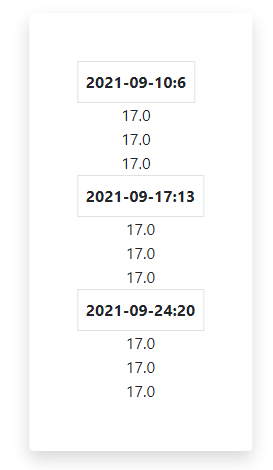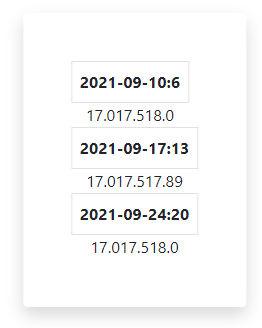# Mapping Array to Table Issue

Hello All,

Trying to map an array to table rows using the following bit of code:

``````strikes = [['17.0','17.5','18.0'],['17.0','17.5','17.89'],['17.0','17.5','18.0']]
``````
``````  tableData() {
let strikes = this.state.strikes;
console.log(strikes)
return strikes.map((key, index) => {
for (let strike in key) {
console.log(key[strike])
return ( <tr>{key[strike]}</tr>);
}
});
}
``````

The thing I am struggling with is why I am only returning the first item of each array value in the table. When I console.log(key[strike]) I get all the values … although repeated.This is how loops work in JavaScript.

In the callback function you have a loop that `return`s the first value. Once that’s done, looping stops, function complete, end.

You’re also mixing up different ways of looping, and using a loop that is specifically for looping over objects rather than arrays, but that can still work, just not like this.

I thought the return is what was stopping the process but couldn’t figure out how to return each row to be rendered.

Just having trouble breaking this down

``````  tableData() {
let strikes = this.state.strikes;
let rows = strikes.map((key, index) => {
for (let strike in key) {
let row = <tr>{key[strike]}</tr>;
}
});
return rows;
}

renderTable() {
let dates = this.state.dates;
return dates.map((key, index) => {
return (
<div>
<th className="border p-2">{key.toUpperCase()}</th>
{this.tableData()}
</div>
);
});
}
``````
``````arr.map(row => <tr>{row.map(col => <td>{col}</td>)}</tr>)
``````

For example

I appreciate the example but am a bit lost.

I am attempting to create a table for each date with the strikes in rows. I want to place these tables in separate divs.

So now I have:

``````table() {
let dates = this.state.dates;
let strikes = this.state.strikes;

return dates.map((key, index) => {
return (
<div>
<th className="border p-2">{key.toUpperCase()}</th>
<tr>{strikes[index]}</tr>
</div>
);
});
}
``````

With the result of:There’s some data missing here, you’ve added another thing on top of the original question (dates array), so can’t tell what it’s supposed to be doing but something like:

``````datesArr.map(date => (
<div>
<table>
{ strikesArr.map(row => (
<tr>
{row.map(col => (
<td>{col}</td>
)}
</tr>
)}
</table>
<div>
))
``````

??

If you want a set of divs, each with a table inside, then you need to specify you want to render a div with a table inside surely? You can’t have a div wrapping a `tr`, that’s not valid HTML

Yes, I left out the table wrapper (doh) … I’m here now:

``````  table() {
let dates = this.state.dates;
let strikes = this.state.strikes;

return dates.map((key, index) => {
return (
<div className="border p-2">
<table>
<th className="border p-2">{key.toUpperCase()}</th>
{strikes[index].map((strike, index) => {
console.log(strike);
<tr><td>{strike}</td></tr>
})}
</table>
</div>
);
});
}

render() {
return <div className="p-5">{this.table()}</div>;
}
}
``````

Now I can see the strikes the right way in the console. But unable to get the rows to display.

Whole Code:

``````class App extends React.Component {
constructor(props) {
super(props);
this.state = {
key: "KOS1RXP2ZUGW0XKIKVNYZBK4ZJAZP5KB",
ticker: "RKT",
strikeCount: "3",
strategy: "ANALYTICAL",
startDate: "2021-08-30",
endDate: "2021-09-30",
dates: [],
strikes: []
//count: 1
};
}

updateTable = () => {

let dates = [];
let strikes = [];

fetch(request)
.then(function (response) {
return response.json();
})
.then(function (data) {
for (let date in Object.getOwnPropertyNames(data.callExpDateMap)) {
dates.push(Object.getOwnPropertyNames(data.callExpDateMap)[date]);
}
for (let key in data.callExpDateMap) {
let strike = data.callExpDateMap[key];
strikes.push(Object.keys(strike));
}
})
.then(
function (data) {
this.setState({
dates: dates,
strikes: strikes
});
}.bind(this)
);
};

componentDidMount() {
this.updateTable();
}

//  componentDidMount() {
//  this.intervalId = setInterval(this.updateTable, 1000);
//}

//componentWillUnmount() {
//  clearInterval(this.intervalId);
//}

table() {
let dates = this.state.dates;
let strikes = this.state.strikes;

return dates.map((key, index) => {
return (
<div className="border p-2">
<table>
<th className="border p-2">{key.toUpperCase()}</th>
{strikes[index].map((strike, index) => {
console.log(strike);
<tr><td>{strike}</td></tr>
})}
</table>
</div>
);
});
}

render() {
return <div className="p-5">{this.table()}</div>;
}
}

ReactDOM.render(<App />, document.getElementById("data"));

``````

Missing`return` will fix it. You’re console logging then not doing anything

1 Like

yep - thank you:

``````  table() {
let dates = this.state.dates;
let strikes = this.state.strikes;

return dates.map((key, index) => {
return (
<div className="border p-2">
<table>
<th className="border p-2">{key.toUpperCase()}</th>
<th className="border p-2">Bid</th>
{strikes[index].map((strike, index) => {
return (
<tr>
<td>{strike}</td>
</tr>
);
})}
</table>
</div>
);
});
}
``````
1 Like

This topic was automatically closed 182 days after the last reply. New replies are no longer allowed.